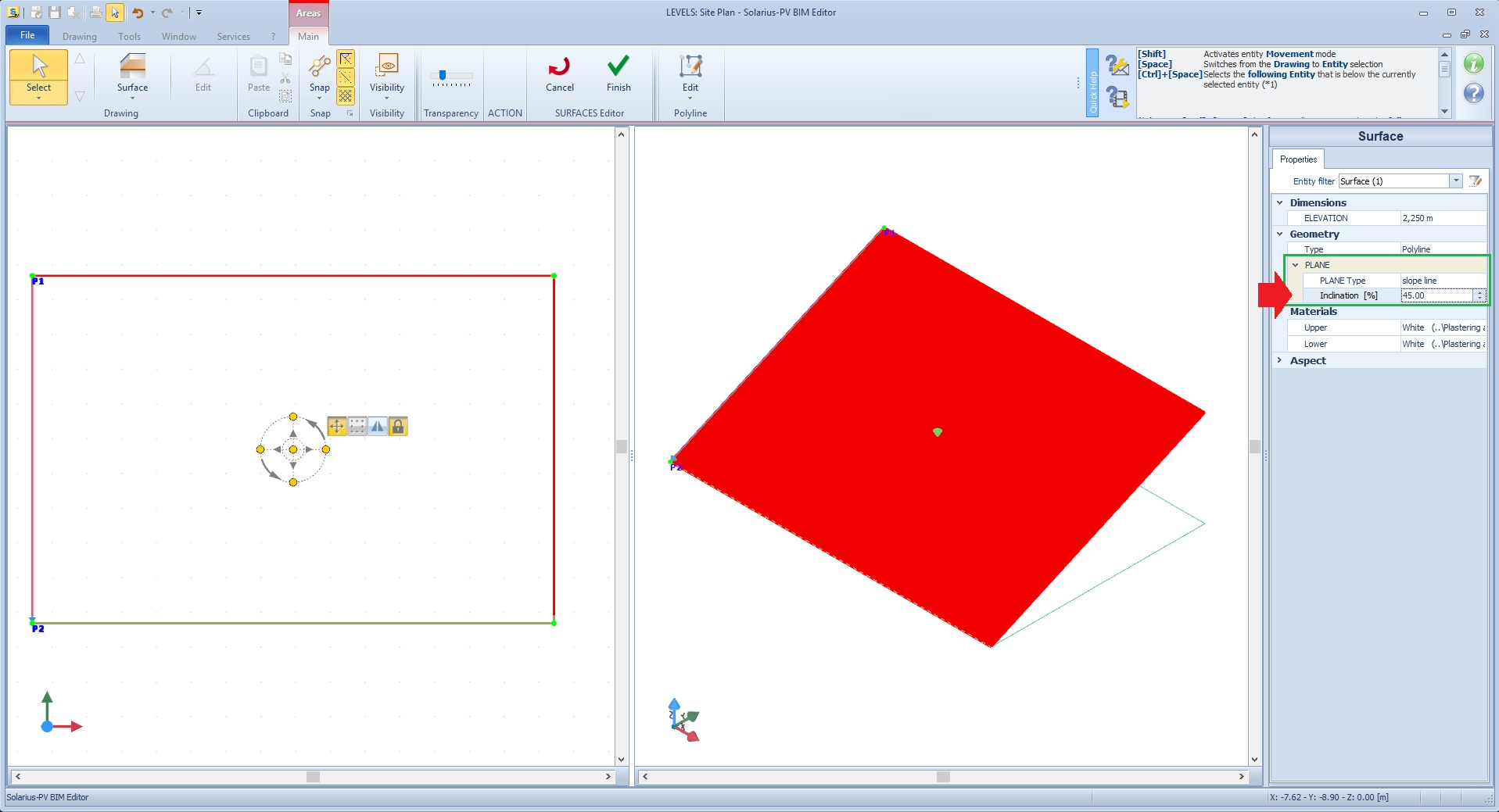# Forum

Helping others, engage in discussions, share your experiences and knowledge with other users.

Vittorio#### How to convert the inclination factor (gradient) from degrees to percentage

Vittorio 2019-07-12 11:28:35

I want to share a request for assistance

Request:
How can I insert the "Inclined surface" Editor, the "Slope [%]" factor requested as a percentage  “%" (See image below), when the data I have is expressed in degrees?
(Ex: roof slab inclination expressed in degrees "°").

You can easily transform to a percentage value, by applying the following formula:

m% = 100*tg ß

where:
- m% - is the "slope" factor required by the software
- ß is the angle expressed in degrees
Conversely, if you have the percentage value and want to convert back to the degrees value, simply proceed with calculating the following inverse formula:

ß=arctang(m%/100)

Ex.01 (conversion from degrees to percentage)
Data: Pitched roof with an inclination angle of 30°:
the gradient factor as a percentage to be is 50.95% (100*tg30).

Ex.02 (conversion from percentage to degrees)
Data: Pitched roof with a percentage inclination of 50.95%: the angle or slope is equal to 30°(arctang (50.95/100)).

N.B. (If using a calculator remember to set it to "DEG" mode)